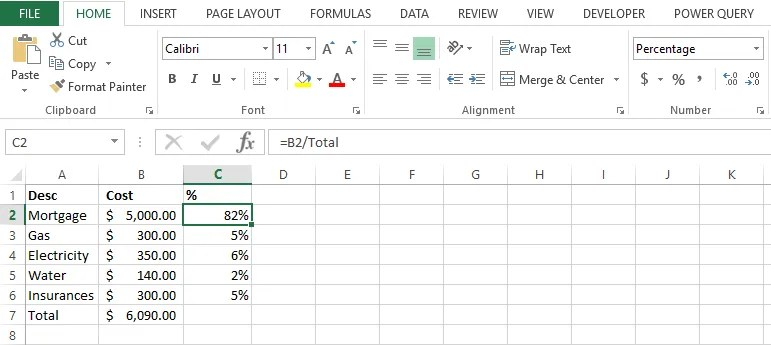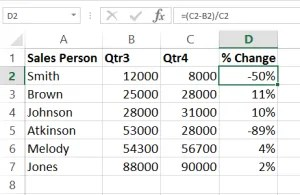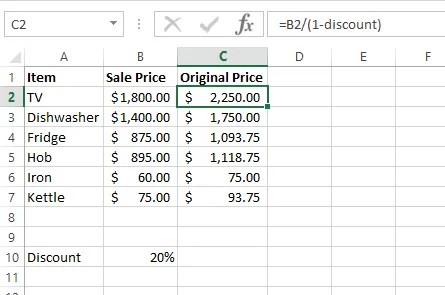# How To Work Out Percentages in Excel

## tax percentages, percentage change, percentage increase, percentage of total….

Whenever I’m delivering an introductory Excel course, one of the core topics I cover is percentages. It’s a basic skill that crops up every day in the business world and is crucial for any Excel user to master. However. the mere mention of the word percentage is often met with users shrinking back in their seats with school day memories of maths coming back to haunt them.

Luckily, you don’t have to fear because if you are using Excel you don’t have to be a mathematical expert and furthermore, with a bit of practise you’ll be churning out percentage formulas in a jiffy!

A technique to make this process even easier is to use named ranges to reference changing cells. Those of you who are familiar with absolute references in formulas will find this useful because it means you don’t have to press F4 to make a cell absolute or type in the \$\$. So here goes…. the first one I get asked about constantly is how to work out the percentage of a value.

## 1. How to Calculate the Percentage of a Value e.g Tax Percentages

A great example of this which most people can relate to of course is GST. How do we calculate the GST on a value?

## Name the Changing Cells to Make Referencing Easy

First we are going to name the cell containing the GST rate . To do this click on the cell (B10 in the example below) and then click into the name box shown in the red circle below. The name box displays the active cell reference. Over type this with a name like GST (spaces are not allowed) and then press Enter.

## Create the Formula to Calculate 10% GST

1. Click into cell C2 and type =
2. Click on cell B2
3. Type * GST
4. Press Enter
5. Drag the bottom right corner of cell C2 (where the small green square is) down to cell C4 to copy the formula down the column
6. Click in cell D2 to calculate the line total
7. Type =
8. Click cell B2
9. Type +
10. Click cell C2
11. Press Enter
12. Drag the bottom right corner of cell D2 (where the small green square is) down to cell D4 to copy the formula down the column

In Excel the finished sheet would look something like this:-## 2. Calculate the Percentage of a Total

In this example I have a list of monthly expenses and a total at the bottom of my list. I want to see each of the individual item prices expressed as a percentage of the overall total.1. I set up the spreadsheet and use AutoSum to total the costs in column B
2. Next up I want to name the Total cell so I click cell B7
3. Click in the Name Box and type Total and press Enter
4. Click cell C2
5. Type =
6. Click B2
7. Type /
8. Type Total
9. Press Enter
10. The formula =B2/Total divides the mortgage cost by the total
11. Click C2 and drag the bottom right corner of the cell to copy the formula down to cell C6
12. The last step is to display the answers as percentages
13. Ensure cells C2 to C6 are selected and click the % icon on the Home tab on the ribbon to display % shown above

## 3. Calculate Percentage Change Between Sales this Quarter and Last Quarter

If you need to calculate the percentage change between two values in Excel, you can use a formula that divides the change itself by the “old” value. A common example would be to calculate the percentage change between sales last quarter and sales this quarter.

How the formula works

(new value – old value) / new value

In the example, the active cell contains this formula:

=(C2-B2)/C21. Set up the sheet and click into cell D2 to calculate the first % change
2. Type =
3. Type (
4. Click C2
5. Type –
6. Click C2
7. Type )
8. Type /
9. Click C2
10. Press Enter
11. Drag the bottom right corner of Cell D2 down to cell D7 to copy the formula
12. The last step is to display the answers as percentages
13. Ensure cells D2 to D7 are selected and click the % icon on the Home tab on the ribbon to display %

## 4. Increase Existing Prices by 15% Without Using a Formula

A question I get asked a lot is how to change an existing price list without having to insert a formula column, just simply change what’s already there. Here’s an easy way to do it using Copy and Paste Special.1. Using my previous example, I now want to add 15% to the values in column B.
2. First of all I type 1.15 into cell E2, this will add 15% on, to add 20% on you would need to type 1.2 and so on
3. Click cell E2 and click Copy or press Ctrl C
4. Select the values you want to increase by 15% in column B
5. Click the drop down button beneath the Paste button just underneath the File menu tab
6. Choose Paste Special
7. Choose Multiply
8. NOTE: you may have to reformat the cells to currency as pasting also copies formats by default
9. Delete the contents of cell E2

## Reverse Calculate Percentages

Reverse calculating can be a useful thing to do in Excel. For example, consider the spreadsheet below, all of the items were marked down by 20% for a huge sale but I need to work out what the original price was before it was marked down.  We can calculate this in Excel by dividing the sale price by 1 minus the discount rate.  See the formula in the top line of the screenshot below.1. Name cell B10 as discount
2. Click in cell C2
3. Type =
4. Click on cell B2
5. Type /(1-discount)
6. Press Enter

Author: Anita Lund owner of Trainers Direct, Microsoft Certified Instructor teaching Excel courses in Sydney